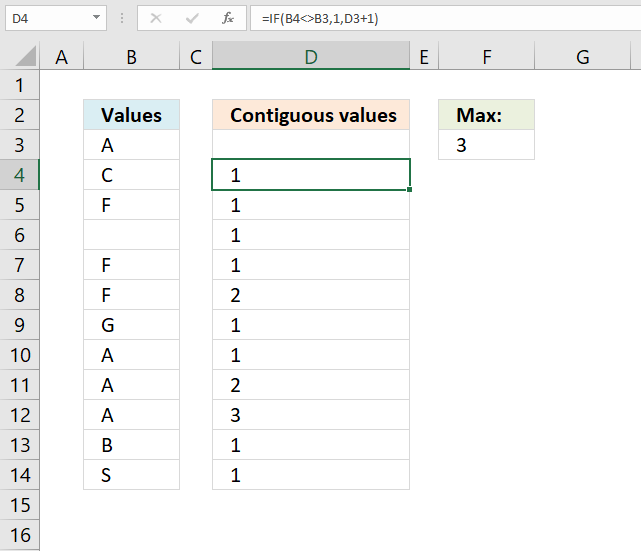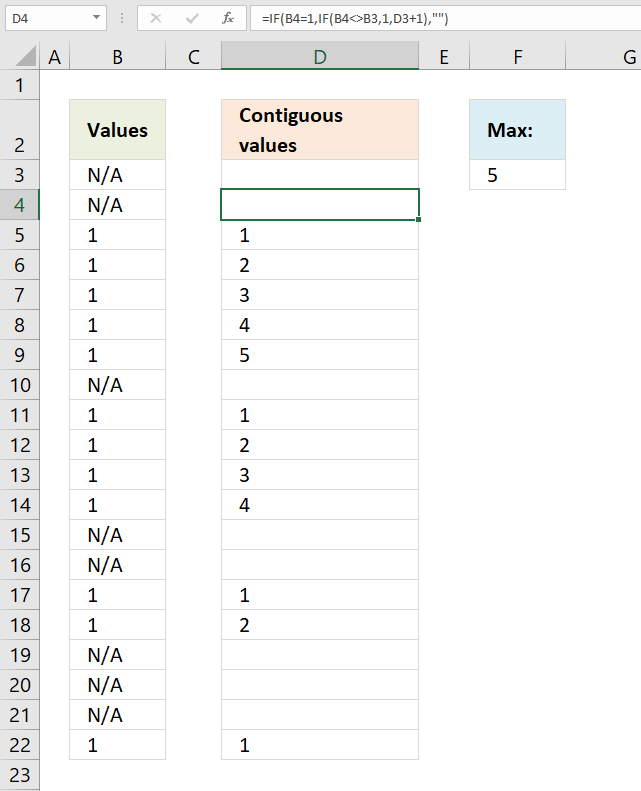Author: Oscar Cronquist Article last updated on April 22, 2021This article demonstrates ways to count contiguous values in a column, in other words, values that repeat and are adjacent.

## 1. How to count any contiguous valueThe formula in cell D4 checks if the value in cell D3 is not equal to the value in cell D4. If true (not equal) the result is 1. If false (equal) the formula adds 1 to the previous value above.

Copy cell D4 and paste to cells below as far as needed in order to count all values in column B.

Formula in cell D4:

=IF(B4<>B3, 1, D3+1)

The formula in cell F3 simply extracts the largest number from cell range D3:D14. Adjust cell ref D3:D14 if you have more values than the example shown in the image above.

Formula in cell F3:

=MAX(D3:D14)

### Explaining formula in cell D4

#### Step 1 - Logical test

Combine the less than character and the larger than character and you get not equal to. The result is a boolean value TRUE or FALSE.

The first argument in the IF function is the logical test, it determines what value to return.

B4<>B3

becomes

"C"<>"A"

and returns TRUE.

#### Step 2 - Evaluate IF function

The IF function returns one value if the logical test returns TRUE and another value if the logical test returns FALSE.

IF(logical_test, [value_if_true], [value_if_false])

IF(B4<>B3, 1, D3+1)

becomes

IF(TRUE, 1, D3+1)

and returns 1 in cell D4.

## 2. How to count a specific contiguous valueHi Oscar,
In column A, I have a long random list of two variables, "N/A" and the value 1. In column B I want to identify the number of contiguous occurrences of the value 1 before the next appearance of "N/A".

I wonder if you could point me to the best way of achieving this, please?

Example below shows what I am after.Much appreciation for your excellent website,
David

Col A Col B
N/A
N/A
1
1
1
1
1 5
N/A
1
1
1
1 4
N/A
N/A
1
1 2
N/A
N/A
N/A
1 1

Thanks, David.

Formula in cell D4:

=IF(B4=1, IF(B4<>B3, 1, D3+1), "")

### Explaining formula in cell D4

#### Step 1 - Logical test

Combine the less than character and the larger than character and you get not equal to. The result is a boolean value TRUE or FALSE.

The first argument in the IF function is the logical test, it determines what value to return.

B4<>B3

becomes

"N/A"<>"N/A"

and returns FALSE.

#### Step 2 - Evaluate IF function

The IF function returns one value if the logical test returns TRUE and another value if the logical test returns FALSE.

IF(logical_test, [value_if_true], [value_if_false])

IF(B4<>B3, 1, D3+1)

becomes

IF(FALSE, 1, D3+1)

becomes

D3+1

becomes

"" + 1

and returns 1.

#### Step 3 - Nested IF function

The second IF function makes sure that the value in cell B4 is equal to 1, we want to only count cells equal to 1.

IF(B4=1, IF(B4<>B3, 1, D3+1), "")

becomes

IF("N/A"=1, IF(B4<>B3, 1, D3+1), "")

becomes

IF(FALSE, IF(B4<>B3, 1, D3+1), "")

becomes

IF(FALSE, 1, "")

and returns "" (nothing) in cell D4.